Select Page# Learn to count with decimals and fractions.

## Calculations with decimal numbers and fractions you will practice in this app.

At school, you learn to make sums with decimals. This is a tricky subject, and many children find this confusing. This app helps children and adults get comfortable with using decimals. You will be able to add or subtract decimals, but also transform decimals into fractions and use them together.
The app, like all MagiWise apps, puts the learning curve as the most important goal. In this app, we focus on numbers with decimals. How do we add them together and subtract them? How do we multiply and divide numbers? How do we use them along with fractions?

These questions will be answered through usage of the app.

The setup is a digital exercise booklet with simple step-by-step instructions and clear exercises. The app contains 32 different lessons with instructions and five tests.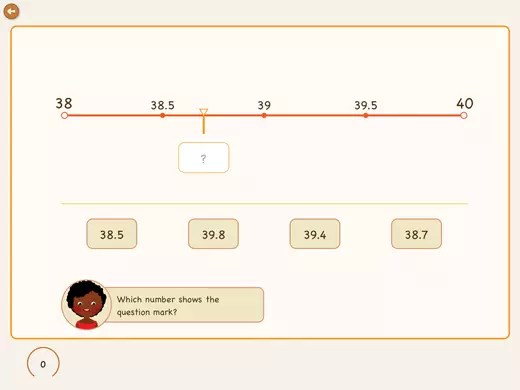### A line with decimals

First, we need to get an understanding of how we create decimals. For example, which tenths are between 1 and 2.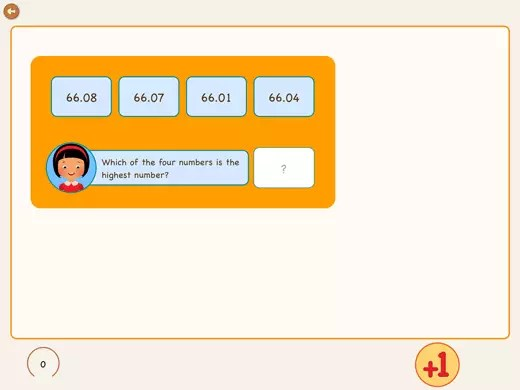### Learn to compare decimals

Which decimals are higher than others? We learn how to recognize the value of a number with tenths and hundredths.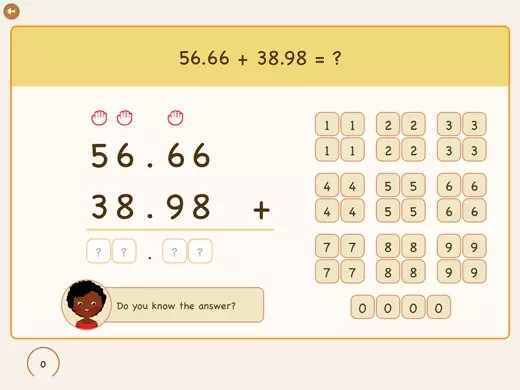The first exercise explains to you how to add decimals together with a line of numbers. The next step is to add them together in conventional sum notation, and finally, you learn to add large numbers with many numerals together.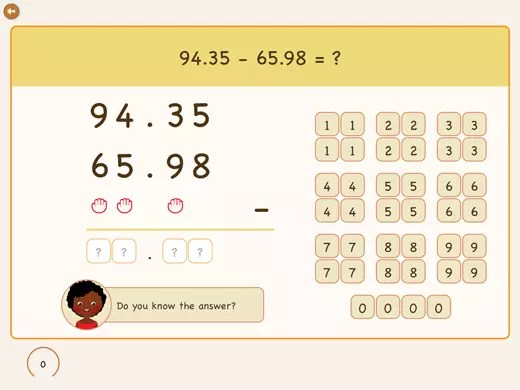### Subtract decimals

After you learn how to add decimals, you will get lessons on how to subtract them from each other. Again, the lessons start with simple challenges but end with numbers with many decimal numerals.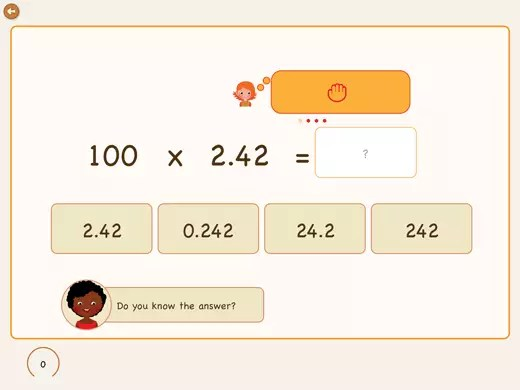### Multiply and divide decimals

In this section, we focus on how to multiply and divide decimals with 10, 100, or 1000. The idea is to get a better understanding of decimals. A decimal represents a tenth, a hundredth, or a thousandth of an integer. It is necessary to understand this concept before we start to mix decimals together with fractions.### Using fractions and decimals together

In this last section, you will learn how to transform a fraction into a decimal. When you are able to convert fractions into decimals, you can quickly add them together or subtract them.

Finally, you can test yourself to see if you understand the full concept of calculating with decimals by solving sums that were exercised during the lessons.

For using the app in the classroom, it is essential to know that the app supports multi-user accounts and works together with the Schoolwork app as well.